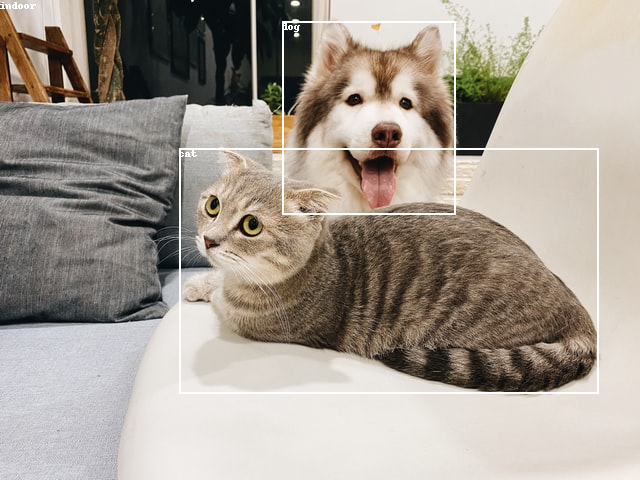# Creating Complex Datasets

Converting a multi-annotation dataset to Deep Lake format is helpful for understanding how to use Deep Lake with rich data.

## How to create datasets with multiple annotation types

#### This tutorial is also available as a Colab Notebook​

Datasets often have multiple labels such as classifications, bounding boxes, segmentations, and others. In order to create an intuitive layout of tensors, it's advisable to create a dataset hierarchy that captures the relationship between the different label types. This can be done with Deep Lake tensor `groups`.
This example show to to use groups to create a dataset containing image classifications of "indoor" and "outdoor", as well as bounding boxes of objects such as "dog" and "cat".

### Create the Deep Lake Dataset

The first step is to download the small dataset below called animals complex.
animals_complex.zip
278KB
Binary
animals complex dataset
The images and their classes are stored in a `classification` folder where the subfolders correspond to the class names. Bounding boxes for object detection are stored in a separate `boxes` subfolder, which also contains a list of class names for object detection in the file `box_names.txt`. In YOLO format, images and annotations are typically matched using a common filename such as `image -> filename.jpeg` and `annotation -> filename.txt` . The data structure for the dataset is shown below:
data_dir
|_classification
|_indoor
|_image1.png
|_image2.png
|_outdoor
|_image3.png
|_image4.png
|_boxes
|_image1.txt
|_image3.txt
|_image3.txt
|_image4.txt
|_classes.txt
Now that you have the data, let's create a Deep Lake Dataset in the `./animals_complex_deeplake` folder by running:
import deeplake
from PIL import Image, ImageDraw
import numpy as np
import os
ds = deeplake.empty('./animals_complex_deeplake') # Create the dataset locally
Next, let's specify the folder paths containing the classification and object detection data. It's also helpful to create a list of all of the image files and class names for classification and object detection tasks.
classification_folder = './animals_complex/classification'
boxes_folder = './animals_complex/boxes'
# List of all class names for classification
class_names = os.listdir(classification_folder)
fn_imgs = []
for dirpath, dirnames, filenames in os.walk(classification_folder):
for filename in filenames:
fn_imgs.append(os.path.join(dirpath, filename))
# List of all class names for object detection
with open(os.path.join(boxes_folder, 'classes.txt'), 'r') as f:
Since annotations in YOLO are typically stored in text files, it's useful to write a helper function that parses the annotation file and returns numpy arrays with the bounding box coordinates and bounding box classes.
"""
Function reads a label.txt YOLO file and returns a numpy array of yolo_boxes
for the box geometry and yolo_labels for the corresponding box labels.
"""
box_f = open(fn)
box_f.close()
# Split each box into a separate lines
lines_split = lines.splitlines()
yolo_boxes = np.zeros((len(lines_split),4))
yolo_labels = np.zeros(len(lines_split))
# Go through each line and parse data
for l, line in enumerate(lines_split):
line_split = line.split()
yolo_boxes[l,:]=np.array((float(line_split), float(line_split), float(line_split), float(line_split)))
yolo_labels[l]=int(line_split)
return yolo_boxes, yolo_labels
Next, let's create the groups and tensors for this data. In order to separate the two annotations, a `boxes` group is created to wrap around the `label` and `bbox` tensors which contains the coordinates and labels for the bounding boxes.
with ds:
# Image
ds.create_tensor('images', htype='image', sample_compression='jpeg')
# Classification
ds.create_tensor('labels', htype='class_label', class_names = class_names)
# Object Detection
ds.create_group('boxes')
ds.boxes.create_tensor('bbox', htype='bbox')
ds.boxes.create_tensor('label', htype='class_label', class_names = class_names_boxes)
# An alternate approach is to use '/' notation, which automatically creates the boxes group
# ds.create_tensor('boxes/bbox', ...)
# ds.create_tensor('boxes/label', ...)
# Define the format of the bounding boxes
ds.boxes.bbox.info.update(coords = {'type': 'fractional', 'mode': 'LTWH'})
In order for Activeloop Platform to correctly visualize the labels, `class_names` must be a list of strings, where the numerical labels correspond to the index of the label in the list.
Finally, let's iterate through all the images in the dataset in order to upload the data in Deep Lake. The first axis of the `boxes.bbox` sample array corresponds to the first-and-only axis of the `boxes.label` sample array (i.e. if there are 3 boxes in an image, the labels array is 3x1 and the boxes array is 3x4).
with ds:
#Iterate through the images
for fn_img in fn_imgs:
img_name = os.path.splitext(os.path.basename(fn_img))
fn_box = img_name+'.txt'
# Get the class number for the classification
label_text = os.path.basename(os.path.dirname(fn_img))
label_num = class_names.index(label_text)
# Get the arrays for the bounding boxes and their classes
# Append data to tensors
'labels': np.uint32(label_num),
'boxes/label': yolo_labels.astype(np.uint32),
'boxes/bbox': yolo_boxes.astype(np.float32)
})

### Inspect the Deep Lake Dataset

Let's check out the second sample from this dataset and visualize the labels.
# Draw bounding boxes and the classfication label for the second image
ind = 1
img = Image.fromarray(ds.images[ind].numpy())
draw = ImageDraw.Draw(img)
(w,h) = img.size
boxes = ds.boxes.bbox[ind].numpy()
for b in range(boxes.shape):
(xc,yc) = (int(boxes[b]*w), int(boxes[b]*h))
(x1,y1) = (int(xc-boxes[b]*w/2), int(yc-boxes[b]*h/2))
(x2,y2) = (int(xc+boxes[b]*w/2), int(yc+boxes[b]*h/2))
draw.rectangle([x1,y1,x2,y2], width=2)
draw.text((x1,y1), ds.boxes.label.info.class_names[ds.boxes.label[ind].numpy()[b]])
draw.text((0,0), ds.labels.info.class_names[ds.labels[ind].numpy()])
# Display the image and its bounding boxes
imgCongrats! You just created a dataset with multiple types of annotations! 🎉